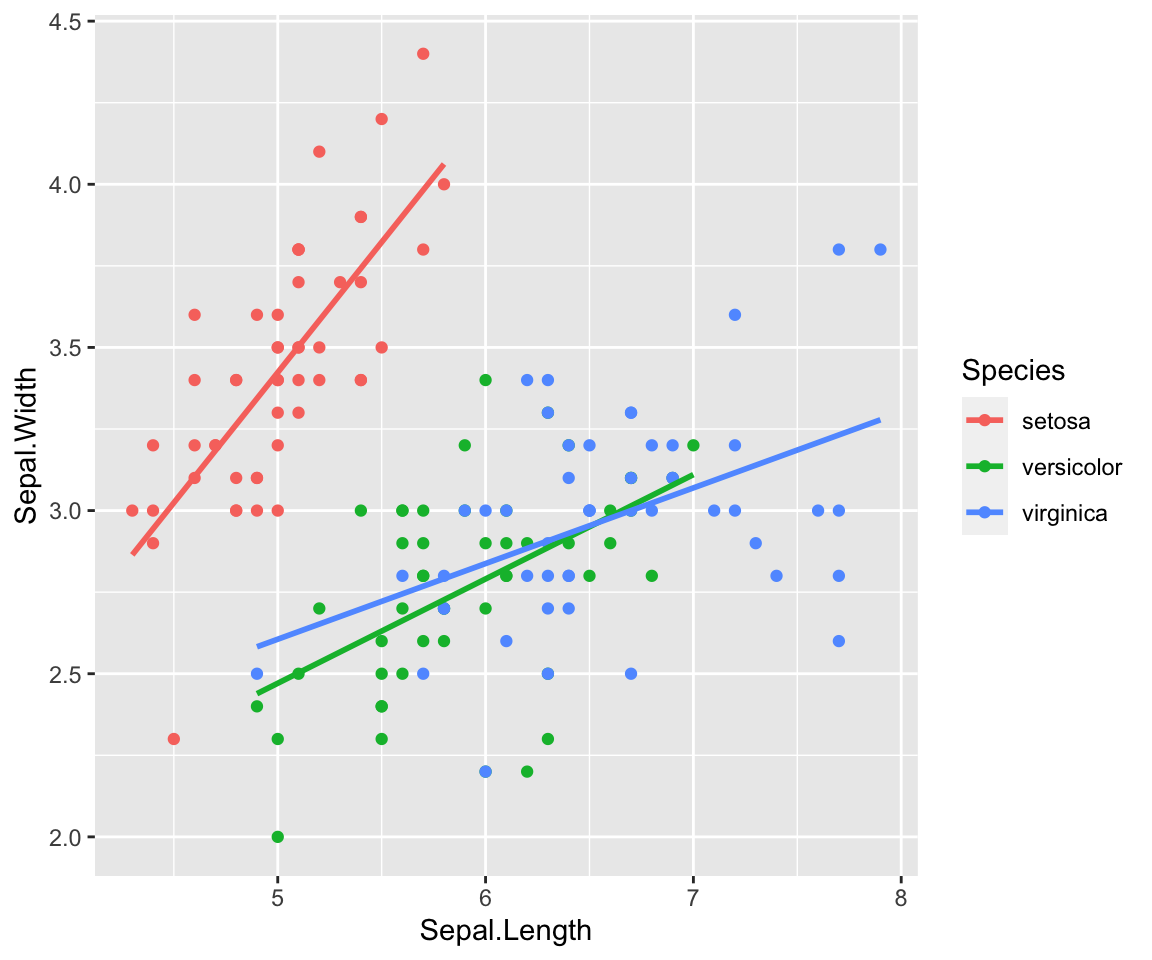You know it, I’m sure: You are developing a data transformation using lots of pipes. Then you need to remove one or more lines. You do this using comments.

So far so good. But if it’s the last line you want to remove a simple comment will produce an error because of the trailing pipe symbol at the end of the second to last line.

Here’s a solution:

## Pipes

### Magrittr

Let’s say you are computing something like this:

 `````` 1 2 3 4 5 6 7 8 9 10 11 12 13 14 15 16 17 18 `````` ``````options(tidyverse.quiet = TRUE) library(tidyverse) options(dplyr.summarise.inform = FALSE) starwars %>% mutate( mean_height = mean(height, na.rm = TRUE) ) %>% group_by(species, gender) %>% summarize( mean_height_species_gender = mean(height, na.rm = TRUE), mean_height = first(mean_height) ) %>% mutate( diff_mean_height = mean_height_species_gender - mean_height ) %>% select(gender, species, diff_mean_height) %>% pivot_wider(names_from = 'gender', values_from = 'diff_mean_height', values_fill = NA) ``````
 `````` 1 2 3 4 5 6 7 8 9 10 11 12 13 14 15 `````` ``````## # A tibble: 38 x 4 ## # Groups: species  ## species masculine feminine `NA` ## ## 1 Aleena -95.4 NA NA ## 2 Besalisk 23.6 NA NA ## 3 Cerean 23.6 NA NA ## 4 Chagrian 21.6 NA NA ## 5 Clawdite NA -6.36 NA ## 6 Droid -34.4 -78.4 NA ## 7 Dug -62.4 NA NA ## 8 Ewok -86.4 NA NA ## 9 Geonosian 8.64 NA NA ## 10 Gungan 34.3 NA NA ## # … with 28 more rows ``````

Now you want to skip the last step pivoting the result. If you just comment out the last line, you’ll get an error:

 `````` 1 2 3 4 5 6 7 8 9 10 11 12 13 14 `````` ``````starwars %>% mutate( mean_height = mean(height, na.rm = TRUE) ) %>% group_by(species, gender) %>% summarize( mean_height_species_gender = mean(height, na.rm = TRUE), mean_height = first(mean_height) ) %>% mutate( diff_mean_height = mean_height_species_gender - mean_height ) %>% select(gender, species, diff_mean_height) %>% # pivot_wider(names_from = 'gender', values_from = 'diff_mean_height', values_fill = NA) ``````
 ``````1 2 3 4 `````` ``````## Error: :15:0: unexpected end of input ## 13: select(gender, species, diff_mean_height) %>% ## 14: # pivot_wider(names_from = 'gender', values_from = 'diff_mean_height', values_fill = NA) ## ^ ``````

But if you use `identity()` as your last step everything is fine:

 `````` 1 2 3 4 5 6 7 8 9 10 11 12 13 14 15 `````` ``````starwars %>% mutate( mean_height = mean(height, na.rm = TRUE) ) %>% group_by(species, gender) %>% summarize( mean_height_species_gender = mean(height, na.rm = TRUE), mean_height = first(mean_height) ) %>% mutate( diff_mean_height = mean_height_species_gender - mean_height ) %>% select(gender, species, diff_mean_height) %>% # pivot_wider(names_from = 'gender', values_from = 'diff_mean_height', values_fill = NA) %>% identity() ``````
 `````` 1 2 3 4 5 6 7 8 9 10 11 12 13 14 15 `````` ``````## # A tibble: 42 x 3 ## # Groups: species  ## gender species diff_mean_height ## ## 1 masculine Aleena -95.4 ## 2 masculine Besalisk 23.6 ## 3 masculine Cerean 23.6 ## 4 masculine Chagrian 21.6 ## 5 feminine Clawdite -6.36 ## 6 feminine Droid -78.4 ## 7 masculine Droid -34.4 ## 8 masculine Dug -62.4 ## 9 masculine Ewok -86.4 ## 10 masculine Geonosian 8.64 ## # … with 32 more rows ``````

### Base R (R >= 4.1.0)

This trick will also work with the new base R pipes:

 `````` 1 2 3 4 5 6 7 8 9 10 11 12 13 14 15 `````` ``````starwars |> mutate( mean_height = mean(height, na.rm = TRUE) ) |> group_by(species, gender) |> summarize( mean_height_species_gender = mean(height, na.rm = TRUE), mean_height = first(mean_height) ) |> mutate( diff_mean_height = mean_height_species_gender - mean_height ) |> select(gender, species, diff_mean_height) |> # pivot_wider(names_from = 'gender', values_from = 'diff_mean_height', values_fill = NA) |> identity() ``````
 `````` 1 2 3 4 5 6 7 8 9 10 11 12 13 14 15 `````` ``````## # A tibble: 42 x 3 ## # Groups: species  ## gender species diff_mean_height ## ## 1 masculine Aleena -95.4 ## 2 masculine Besalisk 23.6 ## 3 masculine Cerean 23.6 ## 4 masculine Chagrian 21.6 ## 5 feminine Clawdite -6.36 ## 6 feminine Droid -78.4 ## 7 masculine Droid -34.4 ## 8 masculine Dug -62.4 ## 9 masculine Ewok -86.4 ## 10 masculine Geonosian 8.64 ## # … with 32 more rows ``````

## ggplot2

The same problem may occur building ggplots:

 ``````1 2 3 4 5 6 `````` ``````iris %>% ggplot(aes(Sepal.Length, Sepal.Width, color = Species, group = Species)) + geom_point() + geom_smooth(method = "lm", se = FALSE, formula = "y ~ x") + # facet_wrap(~Species) + NULL ``````As you can see, I’ve added `NULl` at the end of the ggplot2-pipe. So I comment out the line before without trouble.

## RStudio Plugin breakerofchains

There’s also a plugin for RStudio called breakerofchains. Using this plugin you can set your cursor onto any line of sequence of pipes. When you call the plugin the code is run up to the line your cursor is.